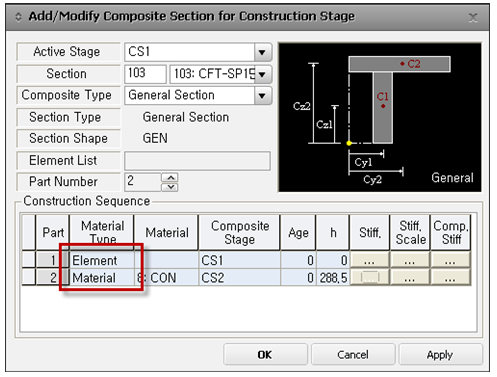Civil Created Edited

# During construction stage analysis of a composite section, the reaction to self-weight appears to be incorrect.

## Question

During construction stage analysis of a composite section, the reaction to self-weight appears to be incorrect.

If the materials of Part 1 and Part 2 are different in a composite section during construction stage definition, the 'Material Type' should be defined as 'Material' instead of 'Element'.

### 1. Composite section for Construction Stage

1) If the cross-section properties are calculated from SPC, and the material type is not defined as 'Material' in the 'Composite section for Construction Stage' in midas Civil, the material information for each part will not be entered. This will result in differences between the calculated cross-section properties from SPC and the results obtained from the midas Civil.

2) In the midas Civil solver, the conversion of cross-sectional area based on the base material defined in SPC is not adjusted by a factor. Instead, the actual cross-sectional properties of each Part are calculated based on the material ratios.

3) During construction stage analysis, section stiffness scale factor / Part stiffness scale factor does not reflect all factors, but only factors related to Asy, Asz, and Ixx. (These are the only factors that are modified at the pre-processing stage and entered into the software.)

### 2.  Construction Sequence1) If the materials of Part 1 and Part 2 are different in a composite section during the construction stage definition, the 'Material Type' should be defined as 'Material' instead of 'Element'. If it is defined as 'Element', Part 1 and Part 2 will be applied as 'Base Material' which is defined in SPC.

### 3.  When defining the construction stages of a composite section, inactive function for self-weight is not supported in any case.

1) If load cases are separated according to the activation stages of each part, self-weight factor can be applied differently. For example, a self-weight factor of 1.5 can be applied to Part 1, and a self-weight factor of 1.2 can be applied to Part 2.

2) 각 Part 별 factor 적용은 가능하나 inactive와 함께 사용하고자 할 때는 inactive가 적용되지 않기 때문에 잘못 사용하는 경우가 발생합니다. 예를 들어 CS1에서 Part 1이 Selfweight Factor=1.5, CS2에서 Part 1의Selfweight Factor=1.0은 적용이 되지 않습니다.

2) Although it is possible to apply factors separately for each part, incorrect usage may occur when attempting to use inactive in conjunction with it, as inactive will not be applied. For example, it is not available that Part 1 has a self-weight factor of 1.5 in CS1 and a self-weight factor of 1.0 in CS2.

0